Courses

# Test: Basic Concepts of Thermodynamics - 2

## 30 Questions MCQ Test RRB JE for Mechanical Engineering | Test: Basic Concepts of Thermodynamics - 2

Description
This mock test of Test: Basic Concepts of Thermodynamics - 2 for Mechanical Engineering helps you for every Mechanical Engineering entrance exam. This contains 30 Multiple Choice Questions for Mechanical Engineering Test: Basic Concepts of Thermodynamics - 2 (mcq) to study with solutions a complete question bank. The solved questions answers in this Test: Basic Concepts of Thermodynamics - 2 quiz give you a good mix of easy questions and tough questions. Mechanical Engineering students definitely take this Test: Basic Concepts of Thermodynamics - 2 exercise for a better result in the exam. You can find other Test: Basic Concepts of Thermodynamics - 2 extra questions, long questions & short questions for Mechanical Engineering on EduRev as well by searching above.
QUESTION: 1

Solution:
QUESTION: 2

### A frictionless piston-cylinder device contains a gas initially at 0.8 MPa and 0.015 m3. It expands quasi-statically at constant temperature to a final volume of 0.030 m3. The work output (in kJ/kg) during this process will be:

Solution:

Iso-thermal work done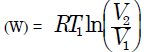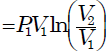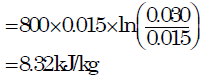QUESTION: 3

### Air is compressed adiabatically in a steady flow process with negligible change in potential and kinetic energy. The Work done in the process is given by:

Solution:

For closed system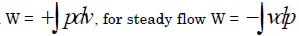QUESTION: 4

A gas expands in a frictionless piston-cylinder arrangement. The expansion process is very slow, and is resisted by an ambient pressure of 100 kPa. During the expansion process, the pressure of the system (gas) remains constant at 300 kPa. The change in volume of the gas is 0.01 m3. The maximum amount of work that could be utilized from the above process is:

Solution:

W = Resistance pressure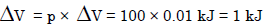this is required for atmosphere, means against atmosphere we have to do this. but question is that how much we can use. Now we use a connecting rod so that it can generate 200 KPa pressure extra. so that total resistance pressure is 300 KPa. now total work done = Total Resistance pressure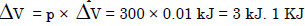for atmosphere. 2 KJ for use

QUESTION: 5

Which of the following variables controls the physical properties of a perfect gas

Solution:
QUESTION: 6

Which of the following is the extensive property of a thermodynamic system?

Solution:
QUESTION: 7

Consider the following:

1. Temperature 2. Viscosity 3. Internal energy 4. Entropy

Which of these are extensive properties?

Solution:

The properties like temperature, viscosity which are Independent of the MASS of the system are called Intensive property

QUESTION: 8

A system comprising a single phase is called a

Solution:
QUESTION: 9

Assertion (A): A thermodynamic system may be considered as a quantity of working substance with which interactions of heat and work are studied.
Reason (R): Energy in the form of work and heat are mutually convertible.

Solution:
•  But remember 100% heat can‟t be convertible to work but 100% work can be converted to heat. It depends on second law of thermodynamics.
•  A thermodynamic system is defined as a definite quantity of matter or a region in space upon which attention is focused in the analysis of a problem.
•  The system is a macroscopically identifiable collection of matter on which we focus our attention
QUESTION: 10

Which of the following is/are reversible process(es)?

1. Isentropic expansion

2. Slow heating of water from a hot source

3. Constant pressure heating of an ideal gas from a constant temperature source

4. Evaporation of a liquid at constant temperature

Select the correct answer using the code given below:

Solution:

Isentropic means reversible adiabatic. Heat transfer in any finite temp difference is irreversible.

QUESTION: 11

Which one of the following represents open thermodynamic system?

Solution:
QUESTION: 12

Hot coffee stored in a well insulated thermos flask is an example of

Solution:
QUESTION: 13

Match List I with List II and select the correct answer using the code given below the lists: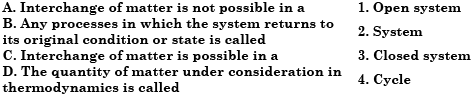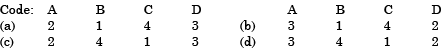Solution:
QUESTION: 14

Consider the following statements:

1. Zeroth law of thermodynamics is related to temperature

2. Entropy is related to first law of thermodynamics

3. Internal energy of an ideal gas is a function of temperature and pressure

4. Van der Waals' equation is related to an ideal gas

Which of the above statements is/are correct?

Solution:

Entropy - related to second law of thermodynamics.
Internal Energy (u) = f (T) only (for an ideal gas)
Van der Wall's equation related to => real gas.

QUESTION: 15

Which one of the following correctly defines 1 K, as per the internationally accepted definition of temperature scale?

Solution:
QUESTION: 16

In a new temperature scale say °ρ, the boiling and freezing points of water at one atmosphere are 100°ρ and 300°ρ respectively. Correlate this scale with the Centigrade scale. The reading of 0°ρ on the Centigrade scale is:

Solution: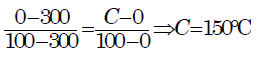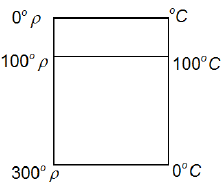QUESTION: 17

Match List-I (Type of Thermometer) with List-II (Thermometric Property) and select the correct answer using the code given below the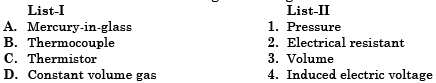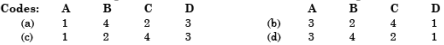Solution:
QUESTION: 18

The time constant of a thermocouple is the time taken to attain:

Solution:

Time Constants: The time constant is the amount of time required for a
thermocouple to indicated 63.2% of step change in temperature of a surrounding media. Some of the factors influencing the measured time constant are sheath wall thickness,
degree of insulation compaction, and distance of junction from the welded cap on an ungrounded thermocouple. In addition, the velocity of a gas past the thermocouple probe greatly influences the time constant measurement. In general, time constants for measurement of gas can be estimated to be ten times as long as those for measurement of liquid. The time constant also varies inversely proportional to the square root of the velocity of the media.

QUESTION: 19

Work transfer between the system and the surroundings

Solution:
QUESTION: 20

There is no work transfer involved in this process

Solution:
QUESTION: 21

The heat transfer, Q, the work done W and the change in internal energy U are all zero in the case of

Solution:

In example of (c), it is a case of free expansion heat transfer, work done, and changes in internal energy are all zero.

QUESTION: 22

Which one of the following is the correct sequence of the three processes A, B and C in the increasing order of the amount of work done by a gas following idealgas expansions by these processes?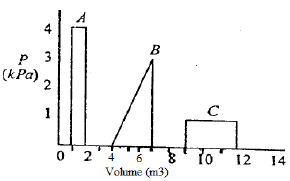Solution: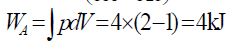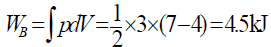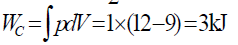QUESTION: 23

A piston cylinder contains air at 600 kPa, 290 K and a volume of 0.01m^3. A constant pressure process gives 54 kJ of work out. Find the final volume of the air.

Solution:

W = ∫ P dV = PΔV
ΔV = W/P = 54/600 = 0.09 m^3
V2 = V1 + ΔV = 0.01 + 0.09 = 0.1 m^3.

QUESTION: 24

Which one of the following expresses the reversible work done by the system (steady flow) between states 1 and 2?

Solution:

For steady flow process, reversible work given by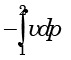QUESTION: 25

The following are examples of some intensive and extensive properties: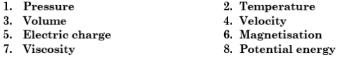Which one of the following sets gives the correct combination of intensive and extensive properties?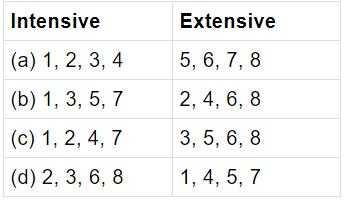Solution:

Intensive properties, i.e. independent of mass are pressure, temperature, velocity and viscosity. Extensive properties, i.e. dependent on mass of system are volume, electriccharge, magnetisation, and potential energy. Thus correct choice is (c). ​

QUESTION: 26

Match List-I with List-II and select the correct answer: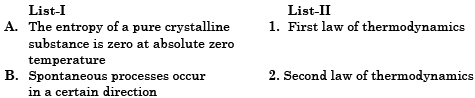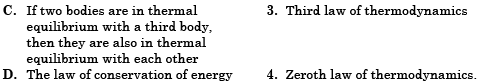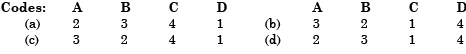Solution:
QUESTION: 27

In free expansion of a gas between two equilibrium states, the work transfer involved

Solution:
QUESTION: 28

In the temperature-entropy diagram of a vapour shown in the given figure, the thermodynamic process shown by the dotted  line AB represents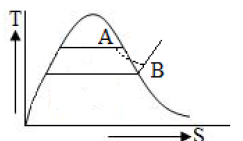Solution:
QUESTION: 29

The door of a running refrigerator inside a room was left open. Which of the following statements is correct?

Solution:
QUESTION: 30

Thermodynamic work is the product of

Solution: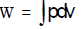where pressure (p) is an intensive property and volume (v) is an extensive property